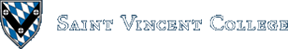Computing & Information Systems    DepartmentSearch CIS Site      Tutorials

## Software Design Using C++

### Introduction

Computers excel at repeating sections of code over and over. They can very quickly do thousands of similar computations, one after the other. (On a computer with more than one processor, several computations may even be able to be done at the same time.) The main way to repeat a section of code in a program is with a "loop" construct. We sometimes speak of looping through a section of code when we are talking about repeating it over and over.

### The FOR Loop

This type of loop is usually used when you know exactly how many times you wish to execute the same section of code. Here is a simple example:

 `````` int k; int sum = 0; for (k = 0; k < 10; k++) { sum = sum + k; cout << sum << endl; } ``````

This loop is executed as follows. First, `k` is initialized to 0. Then a check is made to be sure that `k` is less than 10. (It is.) We then add `k` to `sum` and copy the result back into `sum`. The value of `sum` is then printed on the screen. After going through these 2 lines of code (the "loop body"), the `k++` is executed. This is a quick way to add 1 to a variable. So `k` is now 1. Next we go back to testing the condition to see if `k` is less than 10. Since it is, the loop body is executed again. After that the `k++` increments k to 2, we do the loop body again, etc. The last time through the loop will be when `k` is 9, since 10 is not less than 10. You can probably predict at this point that the output from our little example would be:

 `````` 0 1 3 6 10 15 21 28 36 45 ``````

The general form of a FOR loop is shown below. It has an initialization step, a condition, and an update step, all inside of the parentheses. The statement(s) to be repeated (the loop body) follow this. Note that the loop body is indented by 3 spaces to make it clear what section of code is being repeated. If there is only one statement in the loop body, the curly braces are not needed. If there are two or more statements in the loop body, the braces are necessary so that the statements are seen as a single block. The opening and closing braces should line up vertically, as yet another way to make it clear what section of code is getting repeated. (There are other indenting styles, but the vertical alignment of braces will be used consistently throughout these Web pages.)

 `````` for (INITIALIZATION; CONDITION; UPDATE) LOOP_BODY ``````

The CONDITION is obviously a true/false condition. If the condition is omitted, it is taken as constantly true. The initialization and update steps can also be omitted if desired. The execution of this loop is best summarized in a flow chart (a type of follow-the-arrows diagram):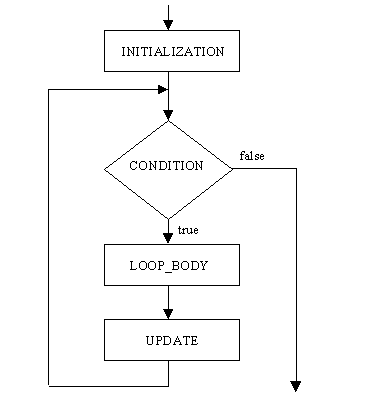The way this works is as follows: First, the INITIALIZATION step is done. Then the CONDITION is checked. If the CONDITION is false, the loop is finished and we proceed to whatever follows the loop. Otherwise, the LOOP_BODY is executed. Always after doing the LOOP_BODY, the UPDATE step is executed. Then we "loop back" and check the CONDITION. If it is false, the loop is over. Otherwise, we do the LOOP_BODY followed by the UPDATE step. This is repeated as needed. If the CONDITION never is false when checked, the loop runs forever!

Although the main use of a FOR loop is in creating a counting-based loop like our example above, other things can be done with this type of loop. As a nonsense example, consider the following:

 `````` for ( ; ; ) cout << "Hello" << endl; ``````

Can you predict what this loop does? If you need help, look at the flow chart. Since the initialization step is empty, the first thing that happens is that the condition is checked. Since that is also empty, it is taken to mean true. Therefore we execute the loop body, printing `Hello` on the screen. Since the update step is empty, nothing happens there. We loop around and try the condition, which is true, of course. So, we again print `Hello`. Since the condition is always true, this goes on forever! We have written what is often called an "infinite loop". Beginning programmers often create infinite loops by accident. If your program runs but seems to produce an infinite stream of output (or seems to be sitting there computing something forever), check your loops to see if you have an infinite loop by mistake.

It's time to look at a complete example. The roots1.cpp program uses a FOR loop to count from 1 to 20, each time printing the number and its square root. Notice that the program sets up a constant named `Max` for the 20. A constant contains a value that cannot be changed. These are highly recommended for values that do not change throughout the course of a program. Then if you should need to create a new version of the program that uses a different constant, you only need to change it in one place, at the top of the program. There is no need to search through the file to find all the places where you used that particular number. Of course, in our short example it doesn't matter much whether we use a constant or a literal 20.

Newer compilers may require you to convert the parameter of the sqrt function to a double instead of leaving it as an int. Thus you may need to use something like this:

 `````` sqrt(static_cast(k)) ``````

If you run the above example, you will see that the numbers don't line up nicely into vertical columns. To do that you need to use some fancy formatting as in roots2.cpp. It uses `setw(10)` to set the output width for the next number to 10 characters. It uses `setprecision(5)` to set the number of digits displayed to 5. It also uses `setiosflags(ios::showpoint)` to indicate that the decimal point (and decimal places) should be shown. This causes 4, for example, to be printed as 4.0000 (which has 5 digits) instead of a plain 4 with nothing following it.

This program loops from 1 to 100, displaying the square roots of each. Since each square root is printed on a new line, that is more output than can fit on the screen. If nothing were done to handle it, this output would quickly scroll past, so that the user would only be able to read the last 20 or so lines of the output. Our program handled this problem by placing the following inside of the loop:

 `````` if ((k % Pause == 0) && (k != Max)) { cout << "Press ENTER to continue"; cin.get(); cout << "Number: Square root:" << endl; } ``````

This is a bit tricky! The % sign indicates the mod operator (integer remainder). So, each time around the loop we are checking the remainder when `k` is divided by 20 (the value of `Pause`). The first time around, when `k` is 1, 20 goes zero times into 1, with a remainder of 1, and 1 is not equal to 0. The second time around, when `k` is 2, 20 again goes 0 times into 2, but with a remainder of 2, which is not equal to 0. This goes on in similar fashion until `k` is 20. Here 20 goes once into 20 with a remainder of 0, so the first condition in the `if` is true. The second condition is also true since `k` is not 100 (the value of `Max`). Thus we execute the 3 lines of code inside the braces: We first prompt the user to press ENTER, then use `cin.get()` to get a character from the keyboard (the ENTER essentially in this case), and finally print column headings for the next group of square roots. You should be able to see by now that the next time the remainder will be 0 will be when `k` is 40. That is just right for pausing things after the second group of twenty square roots.

### The WHILE Loop

The WHILE loop repeats a section of code as long as some condition is true. Of course, the condition is only evaluated at a certain point, as will be clear after we draw the flow chart for this type of loop. In outline, a WHILE loop looks like this:

 `````` while (CONDITION) LOOP_BODY ``````

The flow chart is shown below. Note that the condition is evaluated when we first reach the WHILE loop. If the condition is false, the loop is skipped. If the condition is true, the loop body is executed, and we then go back to testing the condition, etc.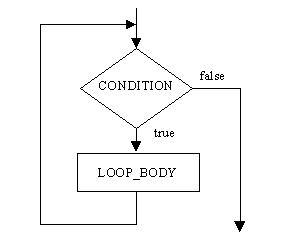The following is a simple example of a WHILE loop. It starts `k` at 1 and doubles it each time around the loop. The number is also printed after being doubled. You should be able to figure out that the numbers printed will be 2, 4, 8, and 16. You might wonder a little about the 16, as that is not less than 10. However, remember that the WHILE condition is only checked at the top of the loop. Thus when `k` is 8, the condition is true. So, we double the value, producing 16, print the 16, and only then loop around and stop because 16 less than 10 is false. At that point control would go on to whatever follows the WHILE loop.

 `````` int k = 1; while (k < 10) { k = 2 * k; cout << k << endl; } ``````

Look at the grade3.cpp program as a more practical example. It prompts the user to repeatedly enter number grades, printing out the corresponding letter grade for each. In order to end the program, the user enters the nonsense grade of -1. The following is an outline of the structure of the WHILE loop used in the main function. Minor details are omitted in order to make the overall structure clearer.

 `````` cin >> NumberGrade; while (NumberGrade != -1) { LetterGrade = ConvertGrade(NumberGrade); cout << "Letter grade is: " << LetterGrade << endl; cin >> NumberGrade; } ``````

Before we reach the loop we read in the first numeric grade. As long as it is not the special ending value -1, we call a function to convert the grade into a letter. That letter grade is then printed on the screen. Before looping around to try the condition again, we read in a new numeric grade so that we test a new value each time around the loop. The only way that the loop will end is if the user enters a -1.

This special -1 value is called a sentinel. A sentinel is any special value used to mark the end of data. The WHILE loop above is just one example of what is called a "sentinel-controlled WHILE loop". This pattern is used so often that it should be memorized so that you do not have to reinvent it. In general the pattern looks like the following, which is written partly in English and partly in C++:

 `````` Get the first data item; while (item != sentinel) { Process the item; Get another data item; } ``````

There are several well-known loop patterns that you should know. See the Programming Patterns section of these Web pages for more information.

In our example program there is a somewhat mysterious use of `cin.get()`. It is used immediately after we read in a numeric grade. This is not absolutely needed in our program. Suppose that the user enters 75 when prompted for a number grade. The 75 goes into the variable, but the newline caused by pressing the ENTER key is read by `cin.get()`. Since the result is not assigned into a variable, printed, or similar, the newline is just thrown away. In our particular program, we could leave out the `cin.get()`. That's because failing to handle the newline does not hurt as long as you are only reading numbers. A newline is not part of any legitimate number, so things will not become confused. The next time the program reads a number grade, the newline will be sitting there. Since it is not part of a number, the program will toss it out and wait for a proper number to arrive. Compare this to what happens in the program shown below in the section on the DO..WHILE loop. There you need to handle the newline since the program reads both numbers and characters.

The above program also uses a function that has both a parameter and a return value. The "Given" section of the comments on the function tell us that the parameter `Num` is being used to pass a number into the function. The "Return" section of the comments tells us that the letter grade is being sent back in the function name. Note the use of the `return` statement in the function code to specify the letter to send back. See the Complex Functions section of these Web pages for more information on such functions. This function also uses an "extended if". See the Decisions, Decisions section of these Web pages for more information on `if` statements.

### The DO..WHILE Loop

This type of loop also repeats something as long as a condition is true. However, the condition is tested after executing the loop body, not before as with a WHILE loop. One consequence of this is that the loop body is always executed at least once in a DO..WHILE loop, but the loop body may be entirely skipped in a WHILE loop. Below we have an outline for a DO..WHILE loop and its flow chart:

 `````` do LOOP_BODY while (CONDITION); ``````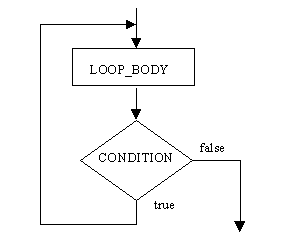A practical example of this type of loop can be found in the grade2.cpp program. The DO..WHILE portion looks like this:

 `````` do { DoOneGrade(); cout << endl << "Do another (y/n)? "; cin >> Reply; cout << endl; } while ((Reply == 'Y') || (Reply == 'y')); ``````

The 4 lines of code in the loop body are repeated as long as `Reply` contains a Y or y character. (Recall that || is the boolean OR operator.) The loop body calls a function to handle everything connected with one grade. Then it explicitly asks the user whether or not to do another grade conversion. The y or n type of answer is read into the character variable `Reply`. At the bottom of the loop, `Reply` is tested to see if it is Y or y.

If you look inside of the `DoOneGrade` function, you will see that it uses a `cin.get()` to read in one character (the newline) after we read in a number grade. In this program this is very important. Without the `cin.get()`, the newline would not be read until we go back to the main function and try to read something. That happens to be the reading of a character into `Reply`. The newline that is already sitting in the input stream would get put into `Reply`, which is not at all what is desired. Things would get quite confused at this point! However, as long as we properly dispose of the newline, we can read a new character into `Reply` with no problem.

You might wonder why we did not read in the newline with `cin.get()` right after reading in a character into `Reply`. That could be done, but is not necessary in this program. That is because the next thing read is a number grade, and when a number is read, any newline already in the input stream gets tossed out anyway as it cannot be part of a number. This is one example that makes it clear that data input in C++ is sometimes fairly low-level and tricky.

### Properties of Loops

The above link gives you a short Web page summarizing the main properties of our three types of loops. It tells you whether the number of times to do the loop body must be known in advance, the minimum number of times that the loop body can be executed, and whether the loop can give an infinite loop.

### Some Dark GDK Examples

Now that we have moved through the topics of conditionals and repetition, we can now create some slightly more interesting Dark GDK Programs. First, let us look at a rather basic 3D application. This program merely creates a cube and moves it back and forth on the screen. For the time being, trust the comments and code regarding how 3D objects are rendered and viewed. The important part for what we are learning is the main GDK event loop:

 `````` while ( LoopGDK ( ) ) { // Move forward / backward depending on the frame multiplier dbMoveObject( 1, frameMultiplier * stepValue ); dbSync ( ); frameNum++; // If frame / 90 yields no remainder, change direction. if(frameNum % 90 == 0) { frameMultiplier *= -1; } } ``````

In this loop, you can see that we will move forward the cube (which has id = 1, used as the first parameter for dbMoveObject) a specified distance. As will be seen in a moment the variable "frameMultiplier" has a value of either 1 or -1 and the variable "stepValue" is 0.5. Therefore, the object is moved either (1 * 0.5 = 0.5) or (-1 * 0.5 = -0.5), depending on the value of "frameMultiplier". After each frame is synchronized, we increment the value of the current "frameNum" and evaluate the next, very important, conditional. The conditional checks to see if (frameNum % 90) equals 0. If we recall from modular arithmetic, this is true for any integer multiple of 90. (e.g. 0, 90, 180, 270). Therefore, on every ninetieth iteration of this loop the frame muliplier is going to be set equal to itself times -1. This means that every 90th iteration, the direction will change for the cube on the next call to dbMoveObject. For a the whole program see CubeMove.cpp. The window of this program should appear like this. NOTE: This will have to be created as a Dark GDK - 3D Game Project.

### 2D Sprite Sheets

As another 2D example, lets look at an application that uses animated sprites. However, before looking at the code, we should look at the basics of sprite sheets. Thus far, we have only utilized single-frame images for our sprites on the screen. Using this methodology, we would have to keep track of multiple sprites if we wanted to use frame-by-frame animation in a program. Luckily for us, Dark GDK allows us to store multiple frames of a sprite in a structure known as a "sprite sheet" which can then be manipulated as though it were a sprite. It is merely a two-dimensional grid of equally-sized rectangles which contain each frame for a given animation. For example, the following 206 x 78 pixel image is a six frame sprite-sheet for a 103 x 26 pixel image: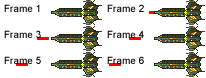When loaded appropriately, this image can be used to define a 6-frame animation of the given ship firing its basic-looking laser. Though the example may appear to be trivial, it utilizes the functions which are generally necessary for using sprite sheets. Let's pull apart the following code:

 `````` // ... Code before dbCreateAnimatedSprite(3, "ShipSpriteSheet.png", 2 , 3, 3); while(LoopGDK()) { dbPlaySprite(3,1,6,200); dbSprite(3,350,275,3); dbSync(); } // ... Code after ``````

The parameters for the "dbCreateAnimatedSprite" function are rather intuitive. The first and last are the SpriteID and ImageID respectively and the second parameter is obviously the relative name of the image used as a sprite sheet. Parameters three and four define the grid size for the given sprite sheet. For our example, we are using a sprite with two columns and three rows. As you can tell, however, the sprite has not been placed on the screen. This is done later with the "dbSprite" function call. However, before that call is "dbPlaySprite" which is used to manage the process of cycling through sprite animations frames. The first parameter is the SpriteID indicating which sprite is to be animated. The next two parameters are the start and end frames for our animation sequence. You need not be bound to the entire sprite sheet when animating. One sprite sheet can contain many various animations, so long as each cell has the same dimensions. The final parameter is the frame-delay in milliseconds. The handling of the animation is done internally within the Dark GDK rendering environment, so this method does not stall the game. Based on previous animation function calls, this will progress the sprite to the appropriate frame after time has elapsed. It must therefore be called each loop to evaluate whether or not the sprite is to be altered. As will be seen in our example, this will allow us to have animations with different delays within the same rendering scene. For an example of such a program, see AnimatedSprite.cpp and download the code with all of its dependencies in AnimatedSprite.zip.

### A Beam Reflecting Program

As another example, let us look at a program which outputs a small line (a "beam") which travels up and down the screen. Each time the beam reaches the edge of the screen, the program plays a cow-bell sound. The code for this program is found in BasicLineBounce.cpp. The bulk of our logic is in the main event loop and follows a finite-state machine approach to the logic managing the movement of the beam. We will only consider it in high-level terms since such machines are the topic of later work in CS 170, Discrete Structures.

A light beam is defined as having one of the following states:

State NameDescription
STARTThis is only valid at the beginning of the program when the light beam begins at the bottom of the screen and "grows" upward until it reaches its full length. Once this is achieved, we enter NORMAL.
NORMALThis is the state of the beam as it makes its way across the bulk of the screen. The state changes here when the "front" of the beam reaches either the top or the bottom of the screen at which point, the state transitions to INCOMING after making adjustments for the change in direction (and playing the cow-bell sound of course).
INCOMINGDuring this state, the front of the beam begins to reverse direction. While in this state, we draw from the rear to the wall. This lasts until the rear of the beam has progressed to exactly the same point as the now-reversed front of the beam. At this point, we transition to OUTGOING.
OUTGOINGFor the rest of this time, we draw from the front to the wall. The rear continues to progress toward the wall while the front moves ahead. When the rear reaches the edge of the wall, we transition back to NORMAL.

In order to compile this application, you will need all of the files in BasicLineBounce.zip.

As one final example, let us look briefly at a simple program which generates a Sierpinski Gasket, which is a type of fractal. Using one method of generating the gasket, namely the "Chaos Game" algorithm, we choose three random, non-linear points in the 2D plane (i.e. we make a triangle). Next, we choose any point within the triangle and call it p. Then, we find the midpoint between p and any one of the three vertices (randomly selected), storing that point in p. After drawing p, we iterate again until we reach our desired count of iterations. This leaves us with this image. The code can be found in Sierpinski.cpp

### Related Item

Programming Patterns

Back to the main page for Software Design Using C++

Author: Br. David Carlson with contributions by Br. Isidore Minerd
Last updated: August 27, 2009
Disclaimer